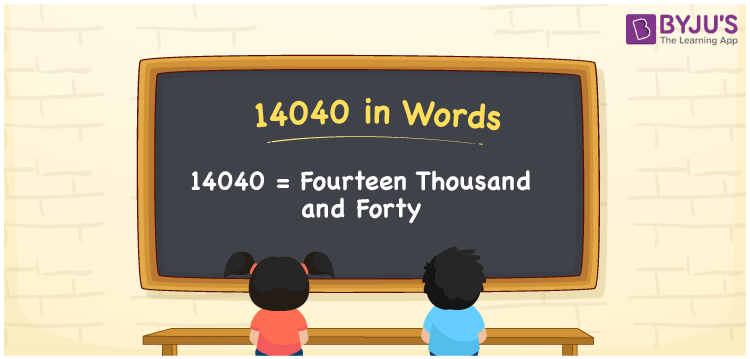# 14040 in Words

The number name of 14040 in words is Fourteen Thousand and Forty. The number 14040 is a cardinal number and it has five digits. For converting any numbers into words, the place value table is found to be useful. In this article, we have discussed the method of writing the number 14040 in words using the place value system in detail.

 14040 in Words: Fourteen Thousand and Forty. Fourteen Thousand and Forty in Numerical Form: 14040.

## 14040 in English Words## How to Write 14040 in Words?

The following table helps you to understand the place values of 14040:

 Ten-Thousands Thousands Hundreds Tens Ones 1 4 0 4 0

The expanded form of 14040 is as follows:

= 1 × Ten thousand + 4 × Thousand + 0 × Hundred + 4 × Ten + 0 × One

= 1 × 10000 + 4 × 1000 + 0 × Hundred + 4 × 10 + 0 × 1

= 10000 + 4000 + 40

= 14040

= fourteen thousand and forty

Hence, 14040 in words is Fourteen thousand and forty.

14040 in words – Fourteen thousand and forty

Is 14040 an odd number? – No

Is 14040 an even number? – Yes

Is 14040 a perfect square number? – No

Is 14040 a perfect cube number? – No

Is 14040 a prime number? – No

Is 14040 a composite number? – Yes

## Frequently Asked Questions on 14040 in Words

Q1

### How to write 14040 in words?

14040 in words is fourteen thousand and forty.

Q2

### Simplify 14000 + 40, and express it in words.

Simplifying 14000 + 40, we get 14040. Hence, 14040 in words is fourteen thousand and forty.

Q3

### Is 14040 a composite number?

Yes, 14040 is a composite number.